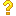•闪存
•博客
• 发言小组
• 投递新闻
• 提问博问
• 添加收藏
• 发布招聘
•文库

2

3

##20编程能力很菜，对于如下代码只能一行一行的写？请大神告知如何用for循环实现？

for (int i = 0; i < k; i ++) { out << DOT << m_p1(i, 0) << " " << m_p1(i, 1) << " " << m_p1(i, 2) <<